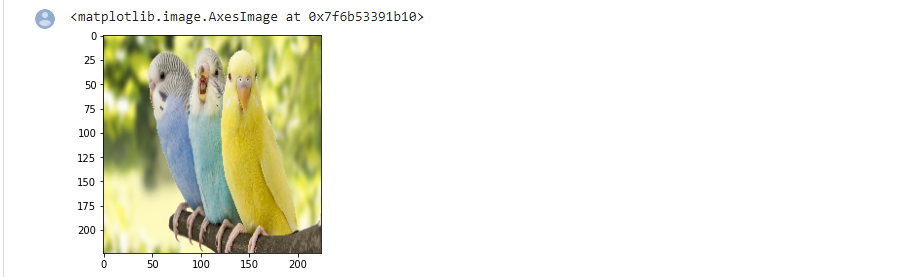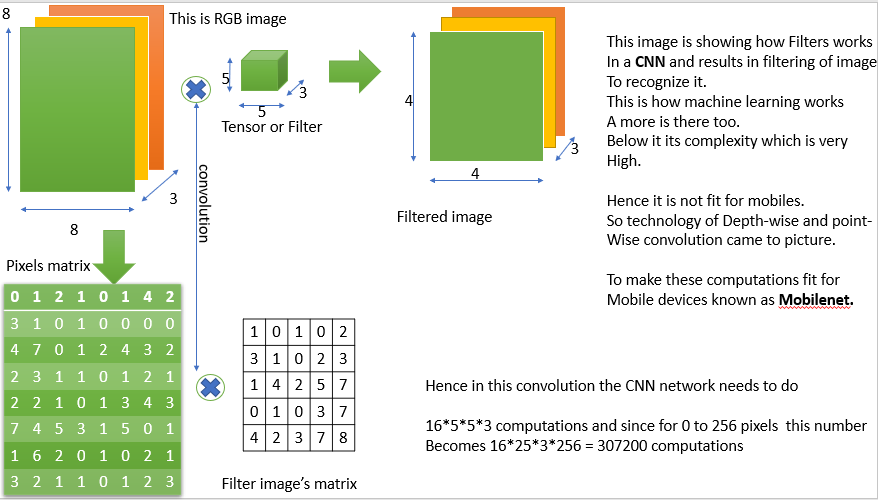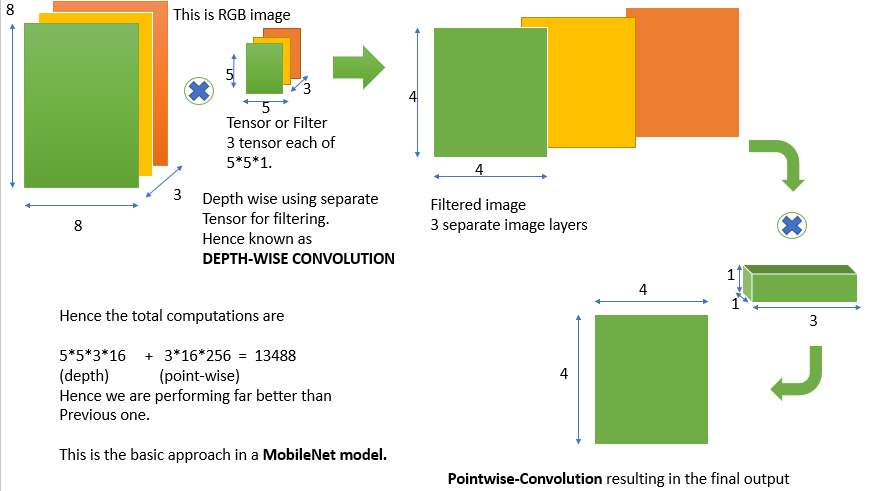Related Articles

# Image Recognition with Mobilenet

• Last Updated : 18 Jul, 2021

Introduction:

Image Recognition plays an important role in many fields like medical disease analysis, and many more. In this article, we will mainly focus on how to Recognize the given image, what is being displayed. We are assuming to have a pre-knowledge of Tensorflow, Keras, Python, MachineLearning

Attention geek! Strengthen your foundations with the Python Programming Foundation Course and learn the basics.

To begin with, your interview preparations Enhance your Data Structures concepts with the Python DS Course. And to begin with your Machine Learning Journey, join the Machine Learning - Basic Level Course

Also, we will be using Colaboratory as our notebook to run python codes and train our models.

Description:

We are aimed to recognize the given image using machine learning. We are assuming we are already having a pre-trained model in our Tensorflow which we will be using to Recognize images. So, we will be using Keras of Tensorflow to import architectures which will help us to recognize images and to predict the image in a better way using coordinates and indexing, we will be using NumPy as a tool.

Steps:

1) At first we have to open Colaboratory and link our Gmail Account to it.

Now at first we will import all the requirements in the notebook and then load our image to be recognised.

import tensorflow as tf

import numpy as np

from tensorflow.keras.preprocessing import image

import matplotlib.pyplot as plt

from tensorflow.keras.applications import imagenet_utils

2) To load the image in the notebook, we have to first add an image file to the folder and then pass its path to any variable (let it be FileName as of now) as:

FileName = ‘Path_to_img’

plt.imshow(img)

Now to display this image we have to load it in our TensorFlow model which can be done using the image library which is present in tensorflow.keras.preprocessing. This library is used to load the image in our model, and then we can print it to display the image as shown below:

In the above method image is displayed in RGB and pixels format by default. So we will be using matplotlib.pyplot to plot our image using coordinates and to get a visualised form of our image in a better way.

The method in lib of matplotlib.pyplot is imshow( image_Variable ) which is used to display image clearly.Hence,Output image

Hence, some of the features we had loaded our image which we are going to recognize.

3) Now we are going to use a pre-trained model which is used to test our predictions on image.

Since there is a large collection of models in tensorflow.keras.applications, so we can use any model to predict the image. Here we will be using mobilenet_v2 model.

Mobilenet_v2 is the 2nd version model of Mobilenet series(although there are many other versions). These models are making use of CNN(Convolutional neural networks) for predicting the features of the images like what is the shape of the object present and what is it matched with.

How CNN works?

Since the images can be seen as a matrix of pixels and each pixel describes some of features of the image, so these technologies uses filters to filter out certain set of pixels in the images and results in the formation of output predictions about images.

CNN uses lot of pre-defined and stored filters and does a convolution (X) of that filter with the pixels matrix of the image. This results in filtering the image’s objects and comparing them with a large set of pre-defined objects to identify a match between them. Hence in this way these models are able to predict the image.CNN’s working.

But these technologies requires a high GPU to increase the comparison rate between millions of data which cannot be provided by any mobile device.

Hence, here comes in action what is known as MobileNet.

Mobilenet is a model which does the same convolution as done by CNN to filter images but in a different way than those done by the previous CNN. It uses the idea of Depth convolution and point convolution which is different from the normal convolution as done by normal CNNs. This increases the efficiency of CNN to predict images and hence they can be able to compete in the mobile systems as well. Since these ways of convolution reduce the comparison and recognition time a lot, so it provides a better response in a very short time and hence we are using them as our image recognition model.Enhancement over the the previous idea

So to import this model in a variable in the model we write the code as :

model = tf.keras.applications.mobilenet_v2.MobileNetV2()

We are now going to feed our loaded image to it in a form of an array, so to convert the image to the array we will use the image library (discussed above) whose method named img_to_array() as given:

Now we are using preprocess_input() and predict() method of our trained dataset to predict image details.

4) Now since the predictions are made, so to display them we have to decode them. To decode them we will be using imagenet_utils. This library is used to decode and make many changes to array images.

A method named decode_predictions( ) is used to decode the predictions made to human-readable format.

results = imagenet_utils.decode_predictions(predictions)# decode_predictions() method is used.

print(results)

Hence, the overall code of the prediction looks like this:

## Python3

 `import` `tensorflow as tf``import` `numpy as np``from` `tensorflow.keras.preprocessing ``import` `image``import` `matplotlib.pyplot as plt``from` `tensorflow.keras.applications ``import` `imagenet_utils``from` `IPython.display ``import` `Image`` ` `# importing image``filename ``=` `'/content/birds.jpg'`` ` `#displaying images``Image(filename,width``=``224``,height``=``224``)``img ``=` `image.load_img(filename,target_size``=``(``224``,``224``))``print``(img)``plt.imshow(img)`` ` `#initializing the model to predict the image details using predefined models.``model ``=` `tf.keras.applications.mobilenet_v2.MobileNetV2()``resizedimg ``=` `image.img_to_array(img)``finalimg ``=` `np.expand_dims(resizedimg,axis``=``0``)``finalimg ``=` `tf.keras.applications.mobilenet_v2.preprocess_input(finalimg)``finalimg.shape``predictions ``=` `model.predict(finalimg)`` ` `# To predict and decode the image details``results ``=` `imagenet_utils.decode_predictions(predictions)``print``(results)`

Output:

We can see the output is containing the name of the bird in the image and the pixels where it is located.

```[[('n01558993', 'robin', 0.8600541), ('n04604644', 'worm_fence', 0.005403478),
('n01806567', 'quail', 0.005208329), ('n01530575', 'brambling', 0.00316776),
('n01824575', 'coucal', 0.001805085)]]```

Hence, we had used Machine Learning Models and Python to Recognize the image of a bird with notebooks.

My Personal Notes arrow_drop_up